Shubham Sachdeva Blog

# Distributing Monte Carlo errors as Blue Noise in Screen Space, Part 2 - Retargeting Pass

In the last part of this series, I talked about the theory presented in  as well talked about how and why we should implement the sorting pass in order to redistribute the seeds using a dithering tile so that we can distribute the monte carlo errors in real time applications as blue noise in screen space. In this part I want to talk about the shortcomings of just using the sorting pass and how we can retarget seeds between frames in order to accumulate and improve our results over frames.

# Sorting Pass Issues

As described in the paper, there are a couple of problems that present themselves when you process by blocks. We want the block size to be a user defined parameter, however, there is no ideal value of the block size and they recommend that we choose a value between 2 and 8. The size of the block size presents us with a tradeoff between the spatio-temporal locality of the input signal, and the descretization of the histogram. If the block size is too large then the spatio-temporal locality assumption has a high chance of being broken as nearby pixels may not be similar at all. On the other hand, if the block size is too small, then the histogram is too coarse and the results will not accumulate over frames. In order to overcome these issues, the authors of the paper created a retargeting pass which accumulates the improvements over frames.

# Retargeting Pass

This figure, as presented in  does a great job of illustrating why the retargeting pass works so well.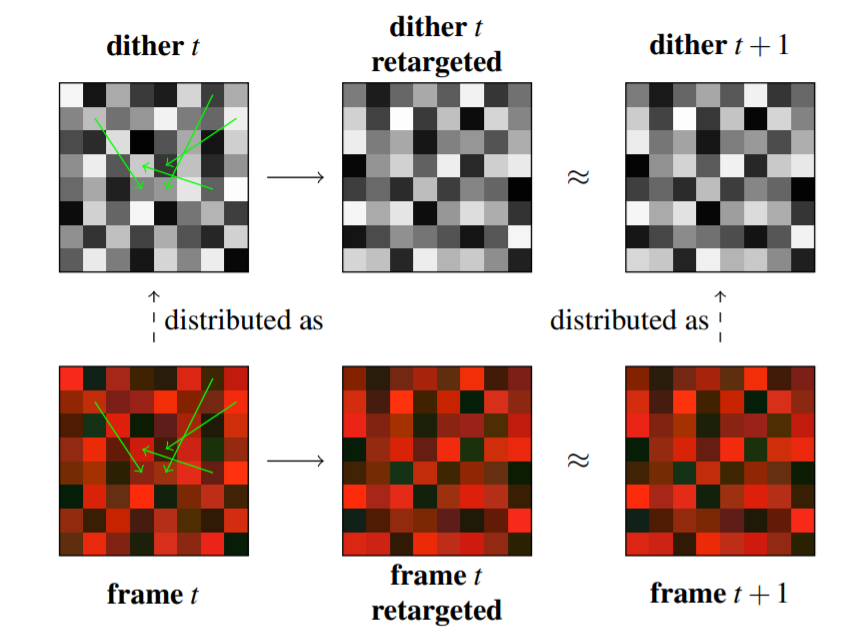The green arrows represent a precomputed permutation which is applied to the seeds. This permutation has been optimized using a process called simulated annealing (see next section) on order to permute the dithering tile of frame x to a tile for frame x+1 so that it can be used for frame x+1. Therefore, if the seeds used to compute the frame x are well distributed as dithering tile x then the retargetted seeds will already be distributed as tile x+1. This way we are optimizing the seeds twice by retargetting the seeds optimized for frame x and then in the sorting pass of frame x+1.

# Precomputing the retargeting pass texture.

In the paper, they describe that they create the retargetted texture using a process described in  called simulated annealing (SA). They start with a tile of frame x and use SA to minimize the difference with tile x+1. In order to accept local permutations they only respect permutations within a radius of 6 and they store the x and y offsets in a 2 channel texture. The paper doesn’t really describe how exactly the algorithm works but essentially SA takes inspiration from a process in metallurgy called annealing. In this process a metal is heated up and cooled slowly in order to remove internal stress and toughen it. Similarly in SA we heat up our initial state and perform permutations randomly, as the temperature of the system goes down the swaps in the dithering tile get better and we get a global optimum value.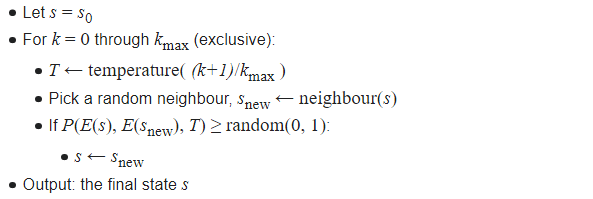This pseudocode from the wikipedia article on Simmulated Annealing does suprisingly well in explaining the process. Note that the energy function (or E(s) in the pseudocode) is the difference between the current tile and the tile of the next frame and when we pick a new neighbour we need to make sure it is less that six units away from the current pixel. In practice instead of storing the actual pixel values in the 2D texture, it is better to store the pixel offsets which will be -6 to 6 in each direction so that retargeting masks of arbitrary sizes can be used.

# Implementation

The retargetting pass itself is extremely simple and can be easily implemented as a compute shader for real time applications. It is placed right after the sorting pass before raytracing the frame. It is important to note that the paper describes tiles and retargeting masks as changing between frames for temporal algorithms, in practice we just use the same tile but just toroidally offset the textures using the R2 sequence described in . Finally in my engine I am adding the blue noise texture in the red channel of the texture and the x and y offsets for retargeting in the green and blue channels respectively. The pseudo-code of the retargeting is as follows but you can also look at the appendix for the actual implementation.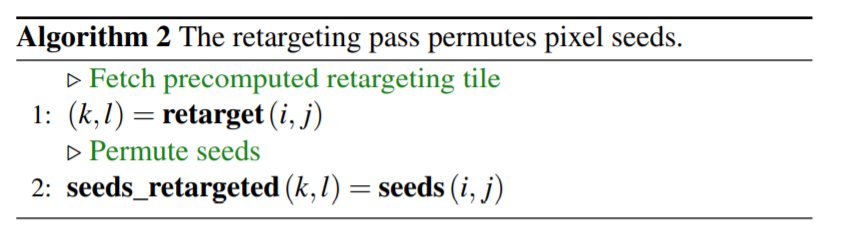# Results

Here is what the scene from part 1 looks like with the retargetting pass added.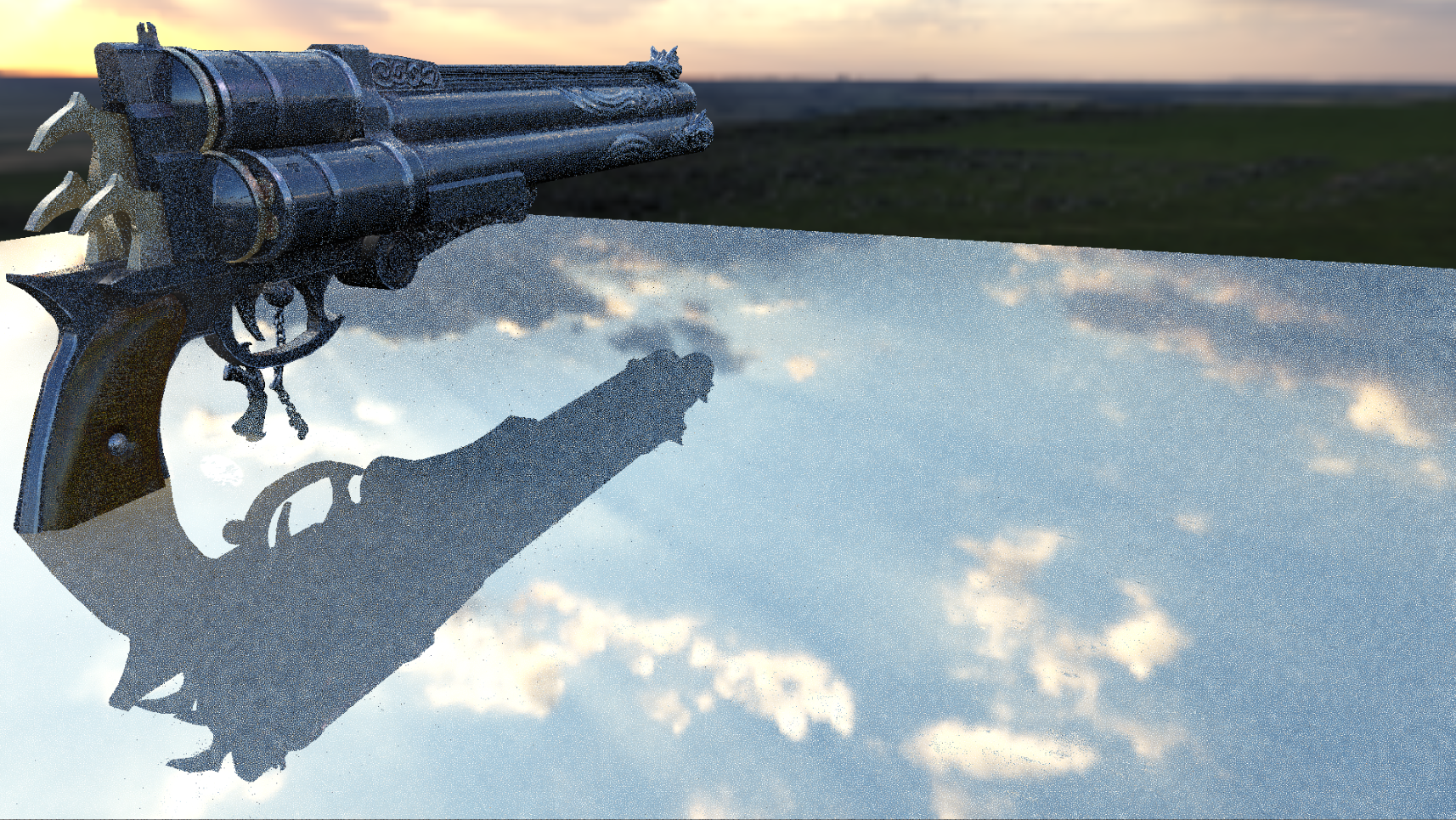Here is also a DFT of the blue noise generated, the results are far superior to what you would have seen by just using the sorting pass.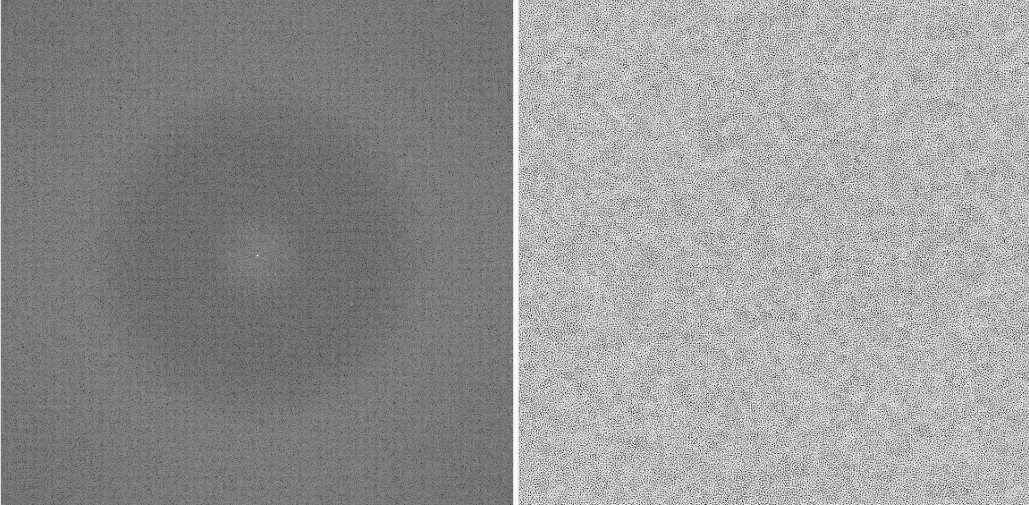# Future Work

As described in the paper, the results presented can be denoised much more easily as compared to white noise. Since the spatiotemporal filters are usually good at removing high frequency details, denoised white noise leaves some low frequency details, however this isn’t an issue with blue noise. In the paper they also mention more improvements to this technique such as permuting only similar pixels so that the sorting pass is more successful even on the edges of the objects. Temporally reprojecting seed values between frames is another improvement in order to accumulate improvements over frames however one critical thing to remember is that that the reprojection should be bijective so that each seed remains unique.

# Appendix - Retartgeting Pass using Compute Shaders

My primary purpose on trying this algorithm was to test its efficacy and potential usability in video games, since that is my main area of interest. As promised here is my compute shader that implements the retargeting pass.

``````[numthreads(4, 4, 1)]
void main(uint3 groupID : SV_GroupID, // 3D index of the thread group in the dispatch.
uint groupIndex : SV_GroupIndex)
{

int texWidth;
int texHeight;

retargetTex.GetDimensions(texWidth, texHeight);

uint samplePosX = (dispatchThreadID.x + offset.x * (texWidth - 1)) % texWidth;
uint samplePosY = (dispatchThreadID.y + offset.y * (texHeight - 1)) % texHeight;

float2 pixelOffsets = retargetTex[uint2(samplePosX, samplePosY)].gb;

pixelOffsets *= 255;

if(pixelOffsets.x > 128)
{
pixelOffsets.x = pixelOffsets.x - 256;
}

if (pixelOffsets.y > 128)
{
pixelOffsets.y = pixelOffsets.y - 256;
}

int2 finalLoc = dispatchThreadID.xy + int2(pixelOffsets.x, pixelOffsets.y);

finalLoc %= uint2(1920, 1080);